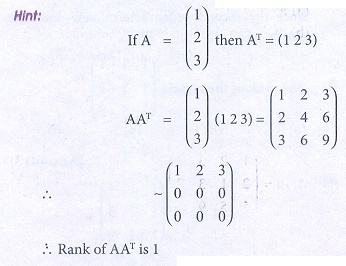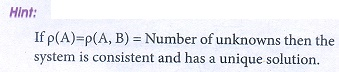Home | | Business Maths 12th Std | Choose the correct answer

## Chapter: 12th Business Maths and Statistics : Chapter 1 : Applications of Matrices and Determinants

Business Maths and Statistics : Applications of Matrices and Determinants: Multiple choice questions with Answers, Solution and Explanation - Book back 1 mark questions and answers with solution for Exercise Problems

1. If A=(1 2 3), then the rank of AAT is

(a) 0

(b) 2

(c) 3

(d) 12. The rank of m û n matrix whose elements are unity is

(a) 0

(b) 1

(c) m

(d) n3. Ifis a transition probability matrix, then at equilibrium A is equal to

(a) 1/4

(b) 1/5

(c) 1/6

(d) 1/84. If A =, then ü(A) is

(a) 0

(b) 1

(c) 2

(d) n5. The rank of the matrixis

(a) 0

(b) 1

(c) 2

(d) 36. The rank of the unit matrix of order n is

(a) n ã1

(b) n

(c) n + 1

(d) n27. If ü (A) = r then which of the following is correct?

(a) all the minors of order r which does not vanish

(b) A has at least one minor of order r which does not vanish

(c) A has at least one (r+1) order minor which vanishes

(d) all (r+1) and higher order minors should not vanish

8. If A =then the rank of AAT is

(a) 0

(b) 1

(c) 2

(d) 39. If the rank of the matrixis 2. Then ö£ is

(a) 1

(b) 2

(c) 3

(d) only real number10. The rank of the diagonal matrix(a) 0

(b) 2

(c) 3

(d) 511. If T =is a transition probability matrix, then the value of x is

(a) 0.2

(b) 0.3

(c) 0.4

(d) 0.712. Which of the following is not an elementary transformation?

(a) Ri ã Rj

(b) Ri ã 2 Ri + 2Cj

(c) Ri ã 2 Ri ã 4Rj

(d) Ci ã Ci + 5Cj13. If ü(A) = ü(A, B) then the system is

(a) Consistent and has infinitely many solutions

(b) Consistent and has a unique solution

(c) Consistent

(d) inconsistent14. If ü(A) = ü(A, B) the number of unknowns, then the system is

(a) Consistent and has infinitely many solutions

(b) Consistent and has a unique solution

(c) inconsistent

(d) consistent15. If ü ( A) ã  ü ( A, B) , then the system is

(a) Consistent and has infinitely many solutions

(b) Consistent and has a unique solution

(c) inconsistent

(d) consistent16. In a transition probability matrix, all the entries are greater than or equal to

(a) 2

(b) 1

(c) 0

(d) 317. If the number of variables in a non- homogeneous system AX = B is n, then the system possesses a unique solution only when

(a) ü ( A ) = ü ( A, B ) > n

(b) ü ( A ) = ü ( A, B ) = n

(c) ü ( A ) = ü ( A, B ) < n

(d) none of these

18. The system of equations 4x + 6 y = 5, 6x + 9 y = 7 has

(a) a unique solution

(b) no solution

(c) infinitely many solutions

(d) none of these19. For the system of equations x + 2 y + 3z = 1, 2x + y + 3z = 2 5x + 5y + 9z = 4

(a) there is only one solution

(b) there exists infinitely many solutions

(c) there is no solution

(d) None of these20. If |A| ã  0, then A is

(a) non- singular matrix

(b) singular matrix

(c) zero matrix

(d) none of these

21. The system of linear equations x + y + z = 2, 2x + y ã z = 3, 3x + 2 y + k = 4 has unique solution, if k is not equal to

(a) 4

(b) 0

(c) ã4

(d) 122. Cramerãs rule is applicable only to get an unique solution when

(a) öz ã  0

(b) öx ã  0

(c) ö ã  0

(d) öy ã  0

23. Ifthen (x y) isAns: (d)24. |An ûn| = 3 |adjA| = 243 then the value n is

(a) 4

(b) 5

(c) 6

(d) 725. Rank of a null matrix is

(a) 0

(b) ã1

(c) ã

(d) 1Tags : Applications of Matrices and Determinants , 12th Business Maths and Statistics : Chapter 1 : Applications of Matrices and Determinants
Study Material, Lecturing Notes, Assignment, Reference, Wiki description explanation, brief detail
12th Business Maths and Statistics : Chapter 1 : Applications of Matrices and Determinants : Choose the correct answer | Applications of Matrices and Determinants